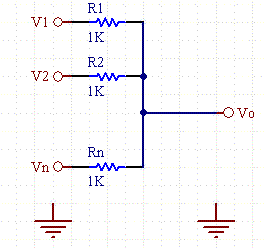Daycounter, Inc. Engineering Services Custom Firmware, Electronics Design, and PCB Layout Home Company Services Products Partners Clients Site Map Contact Us#### Voltage Summer Circuit and CalculatorSee our other Electronics Calculators.

A common analog mathematical computation is to add several voltages:

Vo= V1+ V2.. VN.

This is easily done with the following circuit.Figure 1.  Resistive Adder Circuit Schematic.

Because resistive circuits are linear an analog voltage summer circuit is constructed from one or more resistors combined at a node.  Because of the law of superposition, the output is a sum of the individual input voltages scaled by the resistor networks.  If the resistors are all the same value, then the voltage is the sum of the voltages divided by the number of channels.

The passive circuit results in an attenuation of each signal, so in common practice this circuit is often followed by an active gain element such as an OpAmp to restore the signal levels of each circuit.

 Input Channel Voltage Resistor Channel 1 (V) (Kohms) Channel 2 (V) (Kohms) Channel 3 (V) (Kohms) Channel 4 (V) (Kohms) Channel 5 (V) (Kohms) Channel 6 (V) (Kohms) Channel 7 (V) (Kohms) Channel 8 (V) (Kohms) Vo(1) (V) Vo(2) (V) Vo(3) (V) Vo(4) (V) Vo(5) (V) Vo(6) (V) Vo(7) (V) Vo(8) (V) Vo(sum) (V)

Equations:

Vo= sum( Vn*(Rpn/(Rn+Rpn) ) for n=1 to N

Rpn is the parallel combination of other resistors not including Rn

If all resistors are the same value:

Vo= sum( Vn)/N  for n=1 to N

#### Electronics Manufacturer Links

The best way to find parts is to use our free Electronics Component Directory, where a myriad of electronics manufactures are listed.

#### Other Electronics Links

We offer a broad range of electrical engineering calculators and electronics articles and tutorials

Daycounter specializes in contract electronics design.  Do you need some help on your project?  Contact us to get a quote.